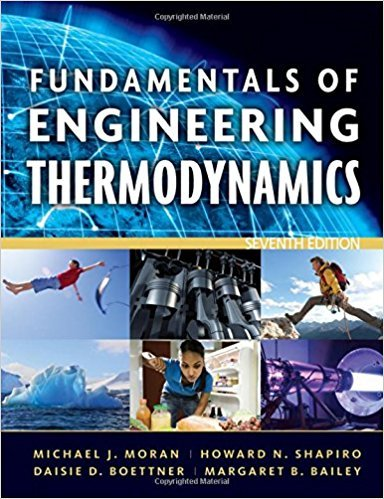×
×

# Solved: Using p ? v ? T DataThe following table listsISBN: 9780470495902 50

## Solution for problem 7P Chapter 3

Fundamentals of Engineering Thermodynamics | 7th Edition

• Textbook Solutions
• 2901 Step-by-step solutions solved by professors and subject experts
• Get 24/7 help from StudySoup virtual teaching assistantsFundamentals of Engineering Thermodynamics | 7th Edition

4 5 0 307 Reviews
31
1
Problem 7P

Using p − v − T Data

The following table lists temperatures and specific volumes of water vapor at two pressures:

 p= 1.0 MPa p=1.5 MPa T (°C) v(m3/kg) T (°C) v(m3/kg) 200 0.2060 200 0.1325 240 0.2275 240 0.1483 280 0.2480 280 0.1627

Data encountered in solving problems often do not fall exactly on the grid of values provided by property tables, and linear interpolation between adjacent table entries becomes necessary. Using the data provided here, estimate

(a) the specific volume at T = 240°C, p = 1.25 MPa, in m3/kg.

(b) the temperature at p = 1.5 MPa, v = 0.1555 m3/kg, in°C.

(c) the specific volume at T = 220°C, p = 1.4 MPa, in m3/kg.

Step-by-Step Solution:

Solution 7P:

Step 1 of 4:-

a)   The specific volume at T = 240°C, P = 1.25 MPa, in m3/kg.

At this temperature, the pressure ofcomes betweenand.

The values of volume in 3rd row of the table are,at.at.The slope of the slanted line will determine the specific volume at the particular pressure..

So, the specific volume atandis:.

Step 2 of 4:-

b) Here we need to determine the temperature at P = 1.5 MPa, V = 0.1555 m3/kg, in°C.

We know the pressure is,.

The volume ofwill be surely in between the temperaturesand. this is evident from the given table.

Diagrammatically,Here also the slope of the slanted line will provide the required temperature.

So,.

So, the temperature at P = 1.5 MPa, V = 0.1555 m3/kg is:.

Step 3 of 4

Step 4 of 4

##### ISBN: 9780470495902

Unlock Textbook Solution